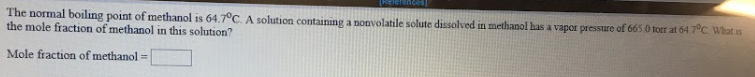# The normal boiling point of methanol is 64.7 degrees C. A solution containing a nonvolatile solute dissolved in methanol has a vapor pressure of 665.0 torr at 64.7 degrees C. Whatis the mole fraction of methanol in this solution? Mole fraction of methanol = ___